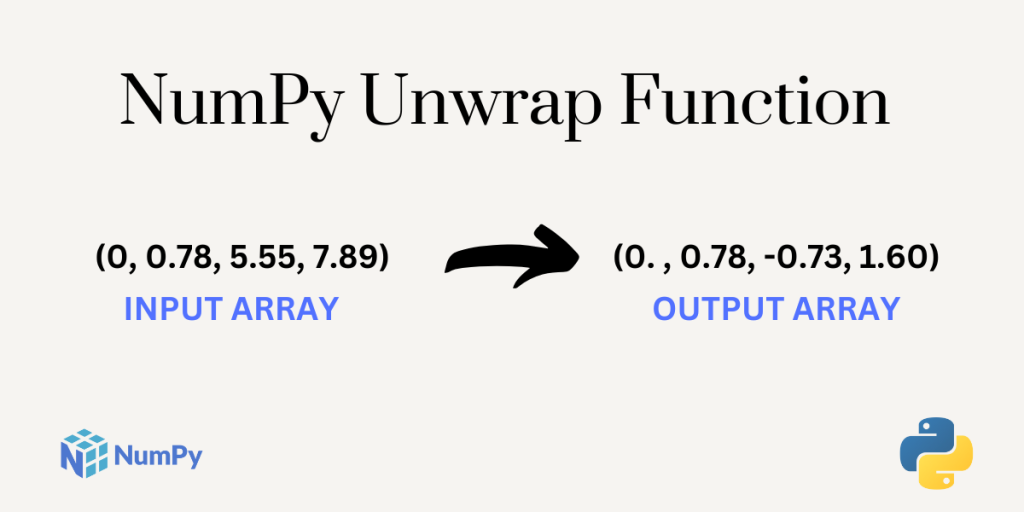# NumPy unwrap – A Complete GuideHello Readers, welcome to another tutorial on NumPy Mathematical Functions. In this tutorial, we will be covering the NumPy unwrap function along with practicing examples. This is an interesting function to understand.

Without any further due, let’s get started.

Also read: NumPy hypot – A Complete Guide

## What is NumPy unwrap?

The `numpy.unwrap()` function is one of the mathematical functions provided by the NumPy library. This function unwraps the given array into a new set of values. In simple words, it transforms the original array of elements into a new set of elements.

Now, let us look at the syntax of the numpy.unwrap() function as it will make things more clear.

### Syntax of NumPy unwrap

```numpy.unwrap ( p,
discont=None,
axis=- 1,
*,
period=6.283185307179586 )
```

The parameters of the function are:

• `p` – It is the input array.
• `discont `– It is the maximum discontinuity between the values of the input array. By default, the `discont` value is pi.
• `axis` – This is an optional parameter. It specifies the axis along which the unwrap function will operate.
• `period` – This parameter is of type float and it’s optional. It represents the size of the input wrap range. The default value is 2*pi.

### Why unwrap an array?

If a NumPy array has discontinuous values then we use the `numpy.unwrap()` function to transform the values of the input array. The unwrap function unwraps or transforms the input array if the jumps between the array elements are greater than the `discont` value of their 2*pi complement value along the given axis.

That’s all you need to catch about the theory of the NumPy unwrap function.

Note: If the discontinuity in the input array is smaller than pi but larger than discont then no unwrapping is done because taking the 2*pi complement would only make the discontinuity larger.

## Working with the NumPy unwrap Function

Let’s get right into the examples of using the arrays

### Default numpy unwrap with no attributes

```import numpy as np

a = np.array((1 , 2 , 3 , 4 , 5))
print("Result1 :\n",np.unwrap(a))

b = np.array((0 , 0.78 , 5.55 , 7.89))
print("Result2 :\n",np.unwrap(b))

```

Output

```Result1 :
[1. 2. 3. 4. 5.]
Result2 :
[ 0.          0.78       -0.73318531  1.60681469]
```

In the above output, you can observe that the input array a has no discontinuity between the elements, and no unwrapping is done for this NumPy array.

In the second output, unwrapping is done when the NumPy array b is passed as an argument to the np.unwrap() function. This is because the discontinuity between 0.78 and 5.55 is greater than the default discount value i.e. pi.

Now, let’s try some more examples where we will set custom values.

### Numpy unwrap with discont attribute

```import numpy as np

a = np.array((5, 7, 10, 14, 19, 25, 32))
print("Result1 :\n",np.unwrap(a , discont=4))

b = np.array((0, 1.34237486723, 4.3453455, 8.134654756, 9.3465456542))
print("Result2 :\n",np.unwrap(b , discont=3.1))
```

Output

```Result1 :
[ 5.          7.         10.          7.71681469  6.43362939  6.15044408
6.86725877]
Result2 :
[0.         1.34237487 4.3453455  1.85146945 3.06336035]
```

For Result1, we have passed a NumPy array `a` as an input to the NumPy unwrap function and the `discont` value is set to 4 i.e. if the jump between the elements of the array a will be greater than or equal to 4 the values will be transformed. Here, the difference between 10 and 14 is 4 hence the values are changed.

For Result2, we have passed a NumPy array b as an input to the NumPy unwrap function and the `discont` value is set to 3.1. Here, the difference between 4.3453455 and 8.134654756 is more than 3.1 and hence the unwrapping is done.

## Summary

That was all about the NumPy unwrap function. The function is really interesting and easy to use. Do check out the NumPy Official Documentation link for some more examples. Stay tuned for some more interesting articles on Python topics 🙂

## Reference

NumPy Documentation – NumPy unwrap